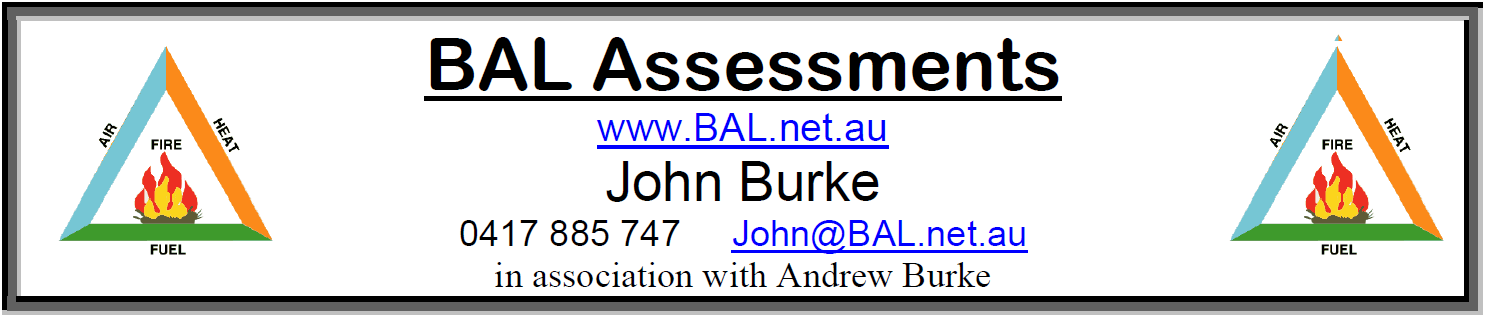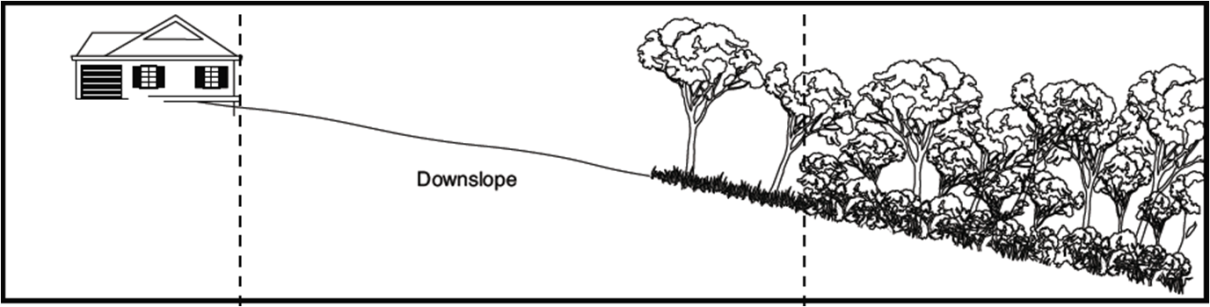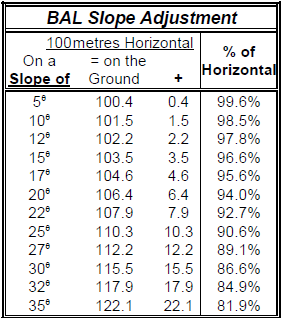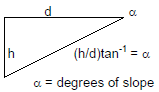© BAL Assessments - How to assess SlopeDetermining the Bushfire Attack Level (BAL)
In accordance with Australian Standard AS 3959

AS3959-2018 requires a horizontal distance of 100 metres to be assessedWe use this simple conversion table for slopes of 10 degrees and over to adjust the measurements
to equate to a horizontal distance as required by the Australian Standard.Try our simple Java distance calculator:
 On Slope Measurement Slope in Degrees Distance on the slope (m) Horizontal Distance (m) Horizontal Measurement Slope in Degrees Horizontal Distance (m) Distance on the slope (m)

We have specific equipment to measure the slope however you might find this formula useful to estimate the slope:10 degree slope is approximately a 1:5.5 height (h) to distance (d) gradient ratio or 18%
15 degree slope is approximately a 1:3.5 height (h) to distance (d) gradient ratio or 28%
20 degree slope is approximately a 1:2.5 height (h) to distance (d) gradient ratio or 40%
30 degree slope is approximately a 1:1.5 height (h) to distance (d) gradient ratio or 60%

Available 7 days a week .... ring us on 0417 885 747

We would welcome the opportunity of expanding on any of the points mentioned above.

BAL ASSESSMENTS
Mobile: 0417 885 747
email: [email protected]
4 | Home | What is a BAL | Bushfire Management Overlay | BAL and Building Costs | What Information do we need | Our Methodology | Our Equipment | Our Assessors | How to assess Slope | Slope Limitations | BAL-Chart |